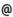## "Babes-Bolyai" University of Cluj-Napoca Faculty of Mathematics and Computer Science

 Galois theory and universal algebras
 Code Semes-ter Hours: C+S+L Credits Type Section MML0006 6 2+1+0 6 optional Matematică MML0006 6 2+1+0 6 optional Matematici aplicate
 Teaching Staff in Charge
 Prof. MARCUS Andrei, Ph.D., marcusmath.ubbcluj.ro
 Prof. PURDEA Ioan, Ph.D., purdeamath.ubbcluj.ro
 Lect. SACAREA Cristian, Ph.D., csacareamath.ubbcluj.ro
 Aims An introduction to Galois theory. The study of notions and basic results of the theory of universal algebras applied to the algebraic structures studied in the previous semesters, completed with new properties.
 Content Galois Theory. Field extensions. Separable extensions and normal extensions. Algebraically closed fields. Finite fields. Wedderburn's theorem. Determination of finite fields and of subfields of a finite field. Galois group. The fundamental theorem of Galois Theory. Solvable groups. Characterization of equations solvable by radicals. Constructibility by ruler and compass. Universal algebras. n-ary operations and universal algebras. Homomorphisms. Stable subsets, subalgebras. The lattice of subalgebras, generated subalgebra. Particular cases: generated subsemigroup, generated subgroup, generated subring, generated submodule. Algebraic closure systems and operators. Direct products of universal algebras. Homomorphic relations. Quotient algebraic congruences. The lattice of congruences. The connection between the congruences of a group and its normal subgroups. The connection between the congruences of a ring and its ideals. Factorization of a homomorphism through a surjective or injective homomorphism. The isomorphism theorems for universal algebras and deduction of the isomorphism theorems for groups and rings.
 References 1. I. PURDEA, G. PIC: Tratat de algebra moderna, Vol.I, Ed. Acad.,1977. 2. I. PURDEA: Tratat de algebra moderna vol. 2, Ed.Acad., 1982. 3. I. PURDEA, I. POP, Algebra, Editura GIL, Zalau, 2003. 4. S. LANG: Algebra, Addison-Wesley, Reading 1965. 5. M.H. FENRICK: Introduction to the Galois Correspondence, 2nd Edition, Birkhauser, Basel 1996. 6. I.D. ION, N. RADU: Algebra. Ed 4. Ed.Didactica si Pedagogica, 1990. 7. I.D. ION, N. RADU, C. NITA, D. POPESCU: Culegere de probleme de algebra, Ed. Didactica si Pedagogica, 1981. 8. M. BALINT, G. CZEDLI, A. SZENDREI: Absztrakt algebrai feladatok, Tankonyvkiado, Budapest 1988. 9. A. MARCUS : Algebra [http://math.ubbcluj.ro/~marcus] 10. L. FUCHS: Algebra, Tankonyvkiado, Budapest 1992. 11. G. SCHEJA, U. STORCH: Lehrbuch der Algebra 1,2, B.G. Teubner, Stuttgart 1994.
 Assessment Homework. Tests (33% x final grade). Oral exam (67% x final grade).